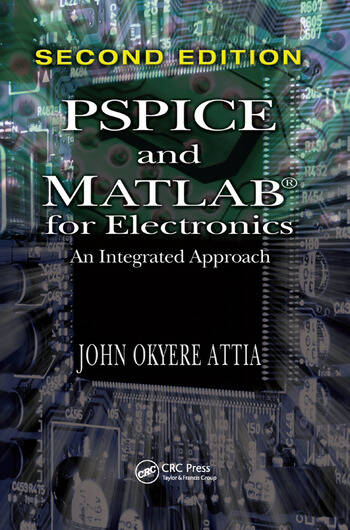# PSPICE and MATLAB for Electronics: An Integrated Approach, Second Edition

## 2nd Edition

John Okyere Attia

CRC Press
Published September 10, 2018
Reference - 382 Pages - 233 B/W Illustrations
ISBN 9781138372740 - CAT# K397593
Series: VLSI Circuits

For Instructors Request Inspection Copy

was \$71.00

USD\$56.80

SAVE ~\$14.20

FREE Standard Shipping!

## Preview

### Summary

Used collectively, PSPICE and MATLAB® are unsurpassed for circuit modeling and data analysis. PSPICE can perform DC, AC, transient, Fourier, temperature, and Monte Carlo analysis of electronic circuits with device models and subsystem subcircuits. MATLAB can then carry out calculations of device parameters, curve fitting, numerical integration, numerical differentiation, statistical analysis, and two- and three-dimensional plots. PSPICE and MATLAB® for Electronics: An Integrated Approach, Second Edition illustrates how to use the strong features of PSPICE and the powerful functions of MATLAB for electronic circuit analysis.

After introducing the basic commands and advanced features of PSPICE as well as ORCAD schematics, the author discusses MATLAB fundamentals and functions. He then describes applications of PSPICE and MATLAB for problem solving. Applications covered include diodes, operational amplifiers, and transistor circuits.

New to the Second Edition

• Updated MATLAB topics
• Schematic capture and text-based PSPICE netlists in several chapters
• New chapter on PSPICE simulation using the ORCAD schematic capture program
• New examples and problems, along with a revised bibliography in each chapter

This second edition continues to provide an introduction to PSPICE and a simple, hands-on overview of MATLAB. It also demonstrates the combined power of PSPICE and MATLAB for solving electronics problems. The book encourages readers to explore the characteristics of semiconductor devices using PSPICE and MATLAB and apply the two software packages for analyzing electronic circuits and systems.

#### Instructors

We provide complimentary e-inspection copies of primary textbooks to instructors considering our books for course adoption.